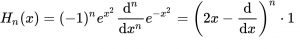Related Articles

# std::hermite in C++

• Last Updated : 18 Jun, 2018

std::hermite is based on the hermite polynomial function given by:After solving the Hermite’s Polynomial, the results come out to be like the following table:

Value of nHermite(n,x)
01
12x
24x2-2
38x3-12x
416x4-48x2+12

Examples:

Input: n = 2 x = 7
Output: 194
Formula for n = 2,
4x7x7 – 2 = 196 – 2 = 194

Input: n = 4 x = 12
Output: 324876
Formula for n = 4,
16x12x12x12x12 – 48x12x12 + 12 = 324876

Syntax:

`std::hermite( unsigned int n, data_type x )`

Parameters: The function accepts two mandatory parameters which are described below:

1. n : Degree of Polynomial
2. x : Value of x to be put in the function. The data_tpye can be float, double or long double.

Return value: This function returns the value which is the answer to the hermite’s polynomial.

Note: The std::hermite() function runs on GCC 7.1 (C++ 17) version.

Below programs illustrate the std::hermite() function:

Program 1:

 `// CPP program to demonstrate the ``// hermite() function``#define __STDCPP_WANT_MATH_SPEC_FUNCS__ 1``#include``int` `main()``{``    ``// spot-checks``    ``std::cout << std::hermite(3, 10) << ``"\n"``;``    ``std::cout << std::hermite(4, 10);``}`

Output:

```7880
15521
```

Error handling

1. If the argument is NaN, NaN is returned and domain error is not reported.
2. If n is greater or equal to 128, the behavior is implementation-defined.

Program 2:

 `// CPP program to demonstrate the ``// hermite() function when n>128 ``#define __STDCPP_WANT_MATH_SPEC_FUNCS__ 1``#include``int` `main()``{``    ``std::cout << std::hermite(129, 10) << ``"\n"``;``}`

Output:

```-2.26912e+149
```
Want to learn from the best curated videos and practice problems, check out the C++ Foundation Course for Basic to Advanced C++ and C++ STL Course for foundation plus STL.  To complete your preparation from learning a language to DS Algo and many more,  please refer Complete Interview Preparation Course.

My Personal Notes arrow_drop_up